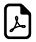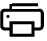# All Math Number Tables and Charts Dynamically#Math Number Tables and Charts Generator

An dynamic and user selection based math tables and charts

Factor,Prime Factor Table  Square Value,Square Root Table

Cube Value,Cube Root Table  Prime Number Table

Even Number Table  Odd Number Table

Power of 2 Table  Power of 3 Table

Power of 5 Table  Log Base(2) Table

Log Base(10) Table  Log Base(e) Table

AntiLog Base(2) Table  AntiLog Base(10) Table

AntiLog Base(e) Table Percentage Table

Sine Table Cosine Table

Tangent Table Cotangent Table##Top Calculators ►